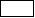# Boundaries of the study domain and modeling strategies

## Introduction

To solve a problem by the finite element method, it is necessary:

• provide the boundaries of the computation domain, i.e. to define the limits or boundaries of the domain
• provide the boundary conditions, i.e. define the values of the state variable (the electric potential ) on the boundaries of the computation domain.

## Application specificity

In the Electric Conduction application only the conducting parts are modeled; air is not taken into account. Thus, it is not necessary to describe the air region adjoining the conducting regions.

## Modeling strategy (3D)

Although it is not necessary to describe the air regions adjoining the conducting regions, the air regions can be represented for reasons detailed afterward. Such regions must be considered as inactive for the electric conduction application (there are no equations to be solved in these regions).

Magnetic flux density computation in the study domain:

The computation of the magnetic flux density B created by the currents flowing within the conducting regions available in the results post-processing phase (an analytic computation by using the Biot-Savart's law). This computation can be carried out in the non-conducting region around the current carrying conductors, if these regions are represented in the Flux geometry.

Linking with a Magneto Static application:

To continue a computation with the Magneto Static application after the computation carried out with the Electric Conduction application, it is necessary that the air areas were represented.

## Boundaries of the study domain with IB

If the technique of the infinite box (IB) is used, then the boundary conditions are automatically assigned by Flux on the infinite box (the electric potential at infinity is the potential of reference set by the user).

## Boundaries of the study domain without IB

If the technique of the infinite box (IB) is not used, the boundary conditions on the border of the computation domain are set by the user by means of the regions known as non-material. The boundary conditions proposed are the following: normal electric field or tangential electric field.

From a practical point of view, to assign the boundary conditions, it is necessary to create “boundary regions”. These are face regions in 3D problems or line regions in 2D problems on the border of the volumes (3D) or on the border of faces (2D) that represent the boundaries of the computation domain.

In the absence of the boundary regions, the default boundary condition is applied. The electric field is tangent to the boundaries.

## Symmetries and periodicities

If symmetries are assigned to the study domain, the user should provide the type of the symmetries. The electric field can be tangent or normal on the symmetry planes defined in the geometry context.

If periodicities are assigned to the study domain, the user should provide the type of the periodicities. Cyclic or anti-cyclic conditions can be imposed on the periodicity planes defined in the geometry context.

## Typical example

A typical example of Electric Conduction problem with an air region around the conductive medium is presented in the figure below.: Regions representing the main parts of the studied device (volume regions in 3D and surface regions in 2D): Regions representing the sources (face regions in 3D and line regions in 2D)